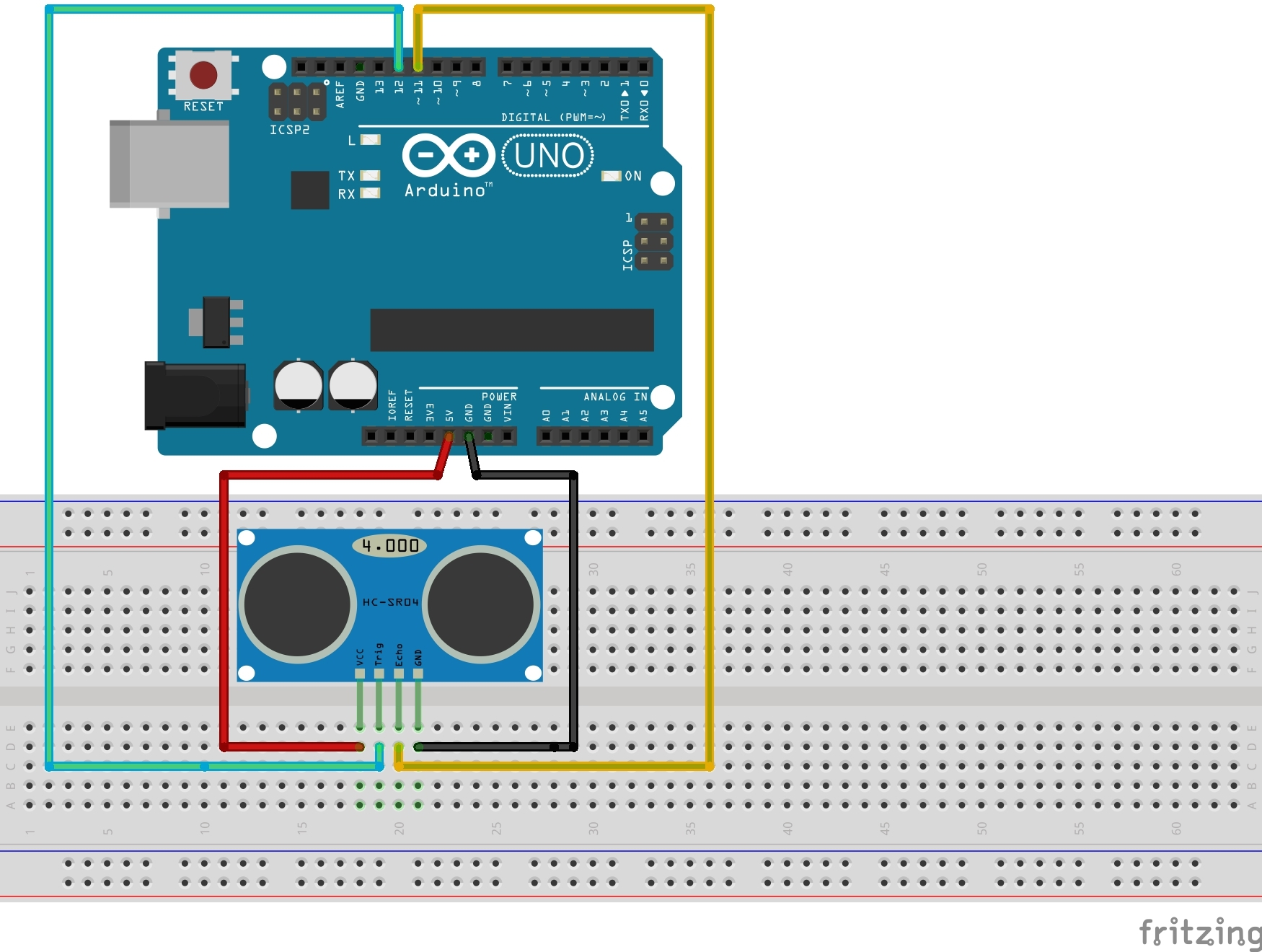# Traffic counter

## Goal

To build a functioning traffic counter. For this purpose we will use an ultrasonic distance sensor. The recorded values ​​will be displayed on the Serial Monitor.

## Materials

• Ultrasonic distance sensor

## Basics

The ultrasonic distance sensor uses sound to calculate the distance between the sensor itself and a given object. The sensor sends out a pulse and measures the time it takes to receive the echo of the pulse. The distance is calculated using the speed of the sound to the object and the measured time.

## Construction

The ultrasonic sensor will be connected to four different ports on the Arduino. For the power supply, connect the VCC pin with the 5V port on the Arduino. To close the circuit, connect the GND pin to the GND port of the Arduino. Finally, connect the echo and the trigger pin of the sensor to two different Arduino digital ports (e.g. 12 and 11).## Programming

Define the pins that are connected to the sensor as usual. In addition to that we need to define two variables to save the measured time and the calculated distance.

int trig = 12; // Trig pin of the sensor is connected to Pin 12
int echo = 11; // Echo-pin of the sensor is connected to Pin 11
unsigned int time = 0;
unsigned int distance = 0;


In setup() we will start the Serial Monitor. We can then define which pins will represent the input and output. The sensor’s trigger pin must be defined as the output and the echo pin will therefore be defined as the input.

Serial.begin(9600);
pinMode(trig, OUTPUT);
pinMode(echo, INPUT);


In loop() we execute a 10 microsecond long ultrasonic pulse:

digitalWrite(trig, HIGH);
delayMicroseconds (10);
digitalWrite(trig, LOW);


The subsequent command time = pulseIn(echo, HIGH); saves the value for the time it takes to receive the echo into the variable time. Finally the distance to the car must be calculated. To do this we use the variable distance. The result we than display on the Serial Monitor.

distance = time / 58;
Serial.println(distance);


For this, you may use a while loop.